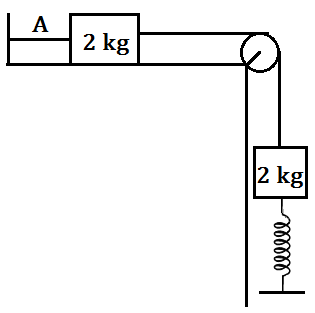# Mechanics Booster-8

At time t=0 string A is cut, then what will be the maximum compression in the spring if spring constant is K.(Assume surfaces are smooth and initially spring was at its natural length)•## 4 Answers

Aditya Agarwal ·

2gk

Niraj kumar Jha ·

(2+√2)gK

Niraj kumar Jha ·

At the mean position, Kx=2g; x=2gK
2gx=12Kx2 +K.E (Using energy conservation)
K.E is the kinetic energy of the two block system ,individually they each have kinetic energy=K.E2
Just after that the string would become loose,So the upper block would continue to move with that velocity,
So at max. compression(h) the kinetic energy of system=K.E2
2gh=12Kh2 +K.E2
Solving we get,h=(2+√2)gK
IS IT CORRECT?

Akash Anand ·

Niraj..I am not able to find the fallacy, but the given answer is far different from this. Given answer is 0.45m .